### IMO Shortlist 2018 problem G4

Kvaliteta:
Avg: 2.0
Težina:
Avg: 7.0

A point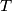$T$ is chosen inside a triangle$ABC$. Let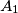$A_1$,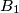$B_1$, and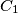$C_1$ be the reflections of$T$ in$BC$,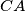$CA$, and$AB$, respectively. Let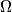$\Omega$ be the circumcircle of the triangle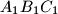$A_1B_1C_1$. The lines$A_1T$,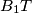$B_1T$, and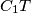$C_1T$ meet$\Omega$ again at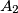$A_2$,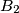$B_2$, and$C_2$, respectively. Prove that the lines$AA_2$,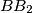$BB_2$, and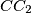$CC_2$ are concurrent on$\Omega$.

Source: https://www.imo-official.org/problems/IMO2018SL.pdf